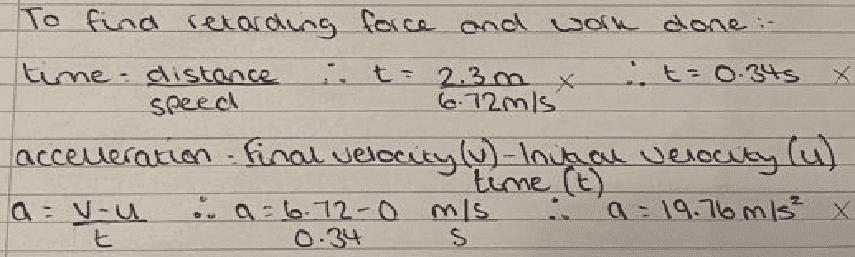# Work, Power and Energy assignment marked wrong somehow? :(

jordanbeaton
Homework Statement:
A press machine has a tooling mass of 42.5kg which falls from a height of 2.3 metres onto an aluminium alloy work piece. If the depth of indentation into the block is 8.25mm calculate the following:
a) KE at impact
b) the impact velocity
c) retarding force on the tool in producing the indentation
d) work done in producing the indentation (assuming the energy is exhausted)
Relevant Equations:
potential energy (PE) = mgh
PE = KE (kinetic energy)
acceleration formula
time formula
velocity break down formula
All of my working out is attached with the photos, I'm struggling to do anymore as the tutor hasn't explained anything like this yet. Any help would be much appreciated

#### Attachments

Cutter Ketch
It goes wrong when you calculate the time. First, what time did you want to calculate? The time to stop the tool, right? You used the velocity at impact, which is fine, but you used the distance it fell which has nothing to do with the deceleration at impact. Even if that had been the right distance, you can’t use d=v t. That is only true if the velocity is constant.

I believe what you want to do here is assume the acceleration is constant. Can you relate constant acceleration, initial velocity, distance, and time?

Homework Helper
Gold MemberThe time to fall is wrong because it does not fall at constant speed. You know ##s=\frac12 a t^2 ## ?
But you don't need this. You've done a, b, now you're looking at the deceleration over the very short distance of the dent.

This will also not be constant speed.
Don't bother to work out the time - you don't need it - and there is an equation that doesn't involve time. Just use the distance, speed and acceleration.